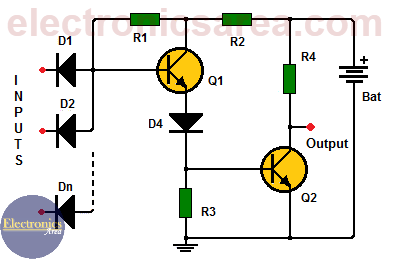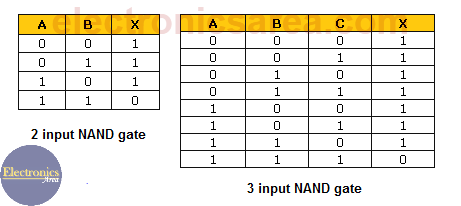NAND gate using transistors

This NAND gate using transistors circuit simulates the operation of a two or more inputs NAND gate. (There is a diode for each input. D1, D2, D3, …, Dn)

How the NAND gate using transistors works?

The truth table below shows the behavior of a NAND gate. We can see that the output is a logic “Low” when all inputs are in “High” logic level.

Any other combination of inputs (“Low” , “High”) will cause the output to have a “High” logic level. The diagram shows that the circuit uses two transistors, working in the cut-off and saturation regions.

How a two input NAND gate using transistors works?

In the case when both inputs are in “High” logic level, the two diodes are inversely polarized and they behave like an open circuit. The transistor Q1 is biased through resistor R1 and enters the saturation region.The saturation current of transistor Q1 biases the Q2 transistor. The Q2 transistor goes into saturation, causing a “Low” logic level at the output (collector Q2).If any or all inputs are logic “Low”, the base of the transistor does not have enough voltage to be polarized and transistor Q1 does not conduct. Without the collector current of Q1, transistor Q2 is not biased, there is no current conduction and the output will be a “high” logic level.Note: The circuit is powered by a 5 volts voltage source.

List of circuit components

• 2  2N2222 NPN bipolar transistors or equivalent (Q1, Q2)
• n  semiconductor diodes 1N914 or equivalent (D1, D2, D3, …. Dn)
• 1  2.2 K resistor (R1)
• 1  470 ohms resistor (R2)
• 1  47 K resistor (R3)
• 1  2.7 K resistor (R4)
• 7
•
•
•
•
•
•
•
•
7
Shares
•
7
Shares
• 7
•
•
•
•
•
•
•
Tagged with: